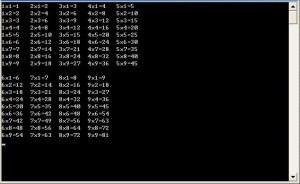# 主控台應用程式寫九九乘法表x為1時，y為1?9；
x為2時，y為1?9；

 ```1 2 3 4 5 6 7 8 9 ``` ``` Dim x, y, result As Integer For x = 1 To 9 For y = 1 To 9 result = x * y Console.WriteLine("{0}x{1}={2}", x, y, result) Next Console.WriteLine("") Next Console.Read()``````1 2 3 4 5 6 7 8 9 10 11 12 13 14 15 16 17 18 19 20 21 22 23 ``` ``` Dim x, y, result As Integer For y = 1 To 9 For x = 1 To 5 result = x * y Console.Write("{0}x{1}={2} ", x, y, result) If result < 10 Then Console.Write(" ") End If Next Console.WriteLine("") Next Console.WriteLine("") For y = 1 To 9 For x = 6 To 9 result = x * y Console.Write("{0}x{1}={2} ", x, y, result) If result < 10 Then Console.Write(" ") End If Next Console.WriteLine("") Next Console.Read()```